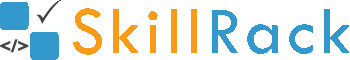Must Solve 100 Programs for Campus Placement Part - 9/104 months ago

1) First Two and Last Two

The program must accept a string S as the input. The program must print the first two characters in S and then print the last two characters in S as the output.

Boundary Condition(s):
4 <= Length of S <= 100

Example Input/Output 1:
Input:
programming

Output:
prng

Example Input/Output 2:
Input:
calculation

Output:
caon

Click the link below to solve this program
http://www.skillrack.com/solve/9214

2) Unit Digit - Ascending and Descending

The program must accept two integers as the input. The program must sort the two integers in ascending order based on their unit digit and print them as the output. If the unit digits are same then sort the two integers in descending order and print them as the output.

Example Input/Output 1:
Input:
57 102

Output:
102 57

Example Input/Output 2:
Input:
275 15

Output:
275 15

Click the link below to solve this program
http://www.skillrack.com/solve/9231

3) First Ten Multiples of X and Y

The program must accept two integers X and Y as the input. The program must print the first 10 multiples of X and Y as the output.

Example Input/Output 1:
Input:
3 7

Output:
3 6 7 9 12 14 15 18 21 24

Example Input/Output 2:
Input:
8 7

Output:
7 8 14 16 21 24 28 32 35 40

Click the link below to solve this program
http://www.skillrack.com/solve/9232

4) Reverse Even Integers

The program must accept N integers as the input. The program must print all the even integers among the N integers in reverse order as the output. If there is no even integer then the program must print -1 as the output.

Boundary Condition(s):
1 <= N <= 100
1 <= Each integer value <= 10^5

Example Input/Output 1:
Input:
5
1 2 5 7 8

Output:
8 2

Example Input/Output 2:
Input:
4
1 3 5 7

Output:
-1

Click the link below to solve this program
http://www.skillrack.com/solve/9233

5) Print Digits - X and Y

The program must accept two integers X and Y as the input. The program must print all the digits from the unit digit of X to the unit digit of Y as the output.

Example Input/Output 1:
Input:
512 69

Output:
2 3 4 5 6 7 8 9

Example Input/Output 2:
Input:
37 44

Output:
7 6 5 4

Click the link below to solve this program
http://www.skillrack.com/solve/9240

6) String without Space

The program must accept a string S with space as the input. The program must print the string without any space as the output.

Boundary Condition(s):
1 <= Length of S <= 100

Example Input/Output 1:
Input:
Hi this is skillrack

Output:
Hithisisskillrack

Example Input/Output 2:
Input:
Incredible India is my country

Output:
IncredibleIndiaismycountry

Click the link below to solve this program
http://www.skillrack.com/solve/9243

7) Count of S2 in S1

The program must accept two string values S1 and S2 as the input where the length of S2 is always 2. The program print the number of occurrences of the string S2 in S1 as the output.

Boundary Condition(s):
2 <= Length of S1 <= 100

Example Input/Output 1:
Input:
tomato
to

Output:
2

Example Input/Output 2:
Input:
AABBBCCBB
BB

Output:
3

Click the link below to solve this program
http://www.skillrack.com/solve/9241

8) Reverse Odd Digits

The program must accept an integer N as the input. The program must print all the odd digits of N in reverse order as the output. If there is no odd digit in N then the program must print -1 as the output.

Example Input/Output 1:
Input:
1047655

Output:
5571

Example Input/Output 2:
Input:
24820

Output:
-1

Click the link below to solve this program
http://www.skillrack.com/solve/9244

9) Inverse Fraction

The program must accept a fraction X/Y as the input. The program must invert the fraction and print the result with the precision up to two decimal places as the output.

Example Input/Output 1:
Input:
3/5

Output:
1.67

Explanation:
3/5 is inverted as 5/3. So the result is 1.67 (with the precision up to 2 decimal places)

Example Input/Output 2:
Input:
5/3

Output:
0.60

Click the link below to solve this program
http://www.skillrack.com/solve/9245

10) Sum - Unit Digit 3 or 6

The program must accept N integers as the input. The program must print the sum of integers having the unit digit as 3 or 6 as the output. If there is no such integer then the program must print -1 as the output.

Boundary Condition(s):
1 <= N <= 100
1 <= Each integer value <= 10^5

Example Input/Output 1:
Input:
5
12 43 30 606 7337

Output:
649

Example Input/Output 2:
Input:
4
52 84 365 134

Output:
-1

Click the link below to solve this program
http://www.skillrack.com/solve/9246